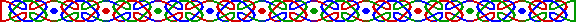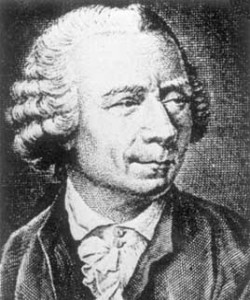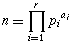(照片取自「The MacTutor History of Mathematics Achieve」http://www-gap.dcs.st-and.ac.uk/~history/ )j(n) = n * (1-p1-1) * (1-p2-1) *.....* (1-pr-1) 。

j(100) = 100 * (1-1/2) * (1-1/5) = 100 * 1/2 * 4/5 = 40。

2*j(n) = j(n+k) 又如何？數學家馬高域斯基 (Andrzej Makowski) 證明對每一個 k 至少有一解。3*j(n) = j(n+k) 又如何？4*j(n) = j(n+k) 又如何？5*j(n) = j(n+k) 又如何？

「非j值」和「非對偶j值」和「歐拉 j函數」一樣：既是解決問題好幫手，亦是產生問題的煩東西。也許數學就是這樣矛盾，也許這才是數學家的腦子「年終無休」。1947 年，基爾 (V. L. Klee) 證明了這猜想對 j(n) < 10100 中所有 n 均成立。1982年 馬沙 ( P. Masai) 及 瓦利特 (A. Valette) 利用同一方法得到 j(n) < 1010000 。1994年，舒拉法 (A. Schlafly) 及 韋根 (S. Wagon) 證明反例的下界在 n > 10^(10^7)。福特 (K. Ford) 把下界推至 n > 10^(10^10)。

Beiler, A. H. Ch. 12 in "Recreations in the Theory of Numbers: The Queen of Mathematics Entertains." New York: Dover, 1966.

Conway, J. H. and Guy, R. K. "Euler's Totient Numbers." The Book of Numbers. New York: Springer-Verlag, pp. 154-156, 1996.

Ribenboim, P. "The Little Book of Bigger Prime" , New York: Springer-Verlag, 1991

Weisstein, E. W. "Totient Function." From MathWorld. http://mathworld.wolfram.com/TotientFunction.html.

Hosted by www.Geocities.ws